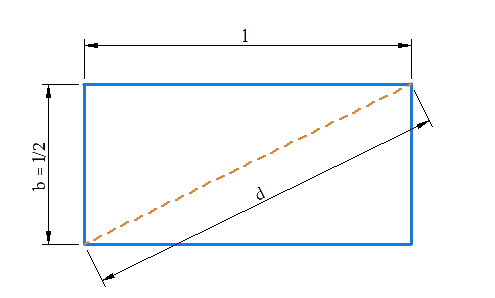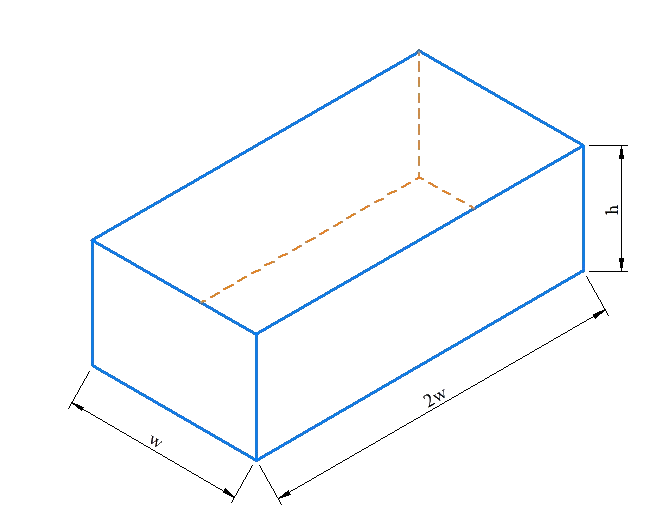# Introduction to the Concept of Functions

Go back to  'Functions'

Let us understand the concept of functions through some examples:

1. The area of a circle can be expressed in terms of its radius $$A = \pi {r^2}$$. The area A is dependent on the radius r. In the language of functions, we say that A is a function of r.

2. The volume V of a sphere is a function of its radius. The dependence of V on r is given by $$V = \frac {4}{3}\pi {r^3}$$.

3. The acceleration a of a body of fixed mass m is a function of the force F applied on the body: $$a = \frac {F}{m}$$.

4. The power P dissipated in a resistor of fixed resistance R is a function of the current I passing through the resistor: $$P = {I^2}R$$.

5. Suppose that a taxi charges a down payment of Rs. 100, and subsequently, Rs. 10 for every kilometer travelled. The taxi fare F is a function of the distance d travelled, and the dependence between the two quantities is given by $$F = 100 + 10d$$ (F is in rupees and d is in kilometers).

Whenever we say that a variable quantity y is a function of a variable quantity x,we mean to say that: y depends on x; the value of y is determined by the value of x. We can write this dependence as follows:

$y = f\left( x \right)$

Observe this notation carefully. It says that: y is a function of x, or depends on x. Do not make the mistake of interpreting the right side as a product (of f and x)! The term $$f\left(x \right)$$ implies a function of x– in other words, a quantity or value which depends on the value of x.

Suppose that y is a function of x. The exact dependence between y and x is that is twice of the square of x. We can write this as follows:

$y = f\left( x \right) = 2{x^2}$

The first half of this relation,$$y = f\left( x \right)$$ tells us that is a function of x, or y depends on x. The second half, $$f\left( x \right) = 2{x^2}$$, tells us the exact definition of this function, i.e., how exactly the function’s output value will be calculated based on the input value. For example,

• When $$x = 1$$, then $$y = f\left( 1 \right) = 2{\left( 1 \right)^2} = 2$$

• When $$x = - 2$$, then $$y = f\left( { - 2} \right) = 2{\left( { - 2} \right)^2} = 8$$

• $$f\left( 3 \right) = 2{\left( 3 \right)^2} = 18$$

• $$f\left( { - \frac{1}{2}} \right) = 2{\left( { - \frac{1}{2}} \right)^2} = \frac{1}{2}$$

Thus, for any value of the input variable (which is x), we can calculate the value of the output variable(which is y), through the relation $$y =f\left( x \right) = 2{x^2}$$.

Example 1:  is a function of x, and the function definition is given as follows:

$y = f\left( x \right) = \frac{1}{{1 + {x^2}}}$

Find the output values of the function for $$x = 0$$, $$x = - 1$$ and $$x = \sqrt 2$$.

Solution: We have:

\begin{align}&f\left( 0 \right) = \frac{1}{{1 +{{\left( 0 \right)}^2}}} = \frac{1}{1} = 1\\&f\left( { - 1} \right) = \frac{1}{{1+ {{\left( { - 1} \right)}^2}}} = \frac{1}{2}\\&f\left( {\sqrt 2 } \right) =\frac{1}{{1 + {{\left( {\sqrt 2 } \right)}^2}}} = \frac{1}{{1 + 2}} =\frac{1}{3}\end{align}

Example 2: The length of a rectangle is twice that of its breadth. Express the area of the rectangle as a function of its (i) length (ii) diagonal length.

Solution: Consider the following figure:(i) In terms of the length l,the area A is

$A = f\left( l \right) = l \times \frac {l}{2} =\frac {{{l^2}}}{2}$

(ii) The diagonal length d is given be

${d^2} = {l^2} + \frac{{{l^2}}}{4} =\frac{{5{l^2}}}{4}$

Thus,

$l = \frac{{2d}}{{\sqrt 5 }},\,\,\,b = \frac{l}{2} =\frac{d}{{\sqrt 5 }}$

Now, the area A can be expressed as a function of d, as follows:

$A = g\left( d \right) = lb = \left({\frac {{2d}}{{\sqrt 5 }}} \right)\left( {\frac{d}{{\sqrt 5 }}} \right) =\frac {{2{d^2}}}{5}$

Note that when expressing A as a function of l, we used the letter f to represent the function.When expressing A as a function of d, we used the letter g.This is because the function definitions are different in the two cases.

Example 3: A rectangular storage container with an open top has a volume of 10 m3. The length of its base is twice its width. Material for the base costs Rs 10 per square meter; material for the sides costs Rs 6  per square meter. Express the cost of materials as a function of the width of the base.

Solution: The following diagram depicts an open container with length and width of the base being 2w and respectively, and its height being h:The area of the base is $$2 w \times w = 2{w^2}$$. Thus, the cost in rupees of the material for the base is.

$10 \times 2{w^2} = 20{w^2}$

Two of the sides have area wh  and the other two have area 2wh, so the cost of the material for the sides is.

$6\left\{ {2\left( {wh} \right) + 2\left( {2wh}\right)} \right\} = 36wh$

The total cost is therefore

$C = 20{w^2} + 36 wh$

To express C as a function of alone, we need to eliminate h, and we do so by using the fact that the volume of the container is 10 m3. Thus,

$\begin{array}{l}\left( {2 w} \right)\left( w\right)\left( h \right) = 10\\ \Rightarrow \,\,\,h =\frac{5}{{{w^2}}}\end{array}$

Substituting this into the expression for C, we have

\begin{align}&C = 20{w^2} + 36 wh\\\,\,\,\,\, &\quad= 20{w^2} + 36 w\left({\frac{5}{{{w^2}}}} \right)\\\,\,\,\,\, &\;\;\;= 20{w^2} +\frac {{180}}{w}\end{align}

This expression gives C as a function of w.

Example 4: A cone has a variable height h and a variable base radius r, but the sum of h and r is fixed. The cone is made of a material of density $$\rho$$. Express the mass of cone as a function of its height h.

Solution: The volume V of a cone is given by:

$V = \frac{1}{3}\pi {r^2}h$

Let the (fixed) sum of h and be k. Thus, $$r = k - h$$, and so:

$V = \frac{1}{3}\pi h{\left( {k - h} \right)^2}$

The mass of the cone can now we expressed as a function of h; m will be $$\rho$$ times the volume V:

$m = f\left( h \right) = \rho V = \frac{1}{3}\pi\rho h{\left( {k - h} \right)^2}$

This is the required function definition.

Functions
Functions
grade 10 | Questions Set 2
Functions
Functions
grade 10 | Questions Set 1